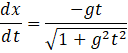1. Science
3. consider a particle that moves along the xaxis with velocity...

# Question: consider a particle that moves along the xaxis with velocity...

###### Question details

Consider a particle that moves along the x-axis with velocity:where g is a constant:

A) Does the particle ever exceed the speed of light? (We are using units in which c = 1)?

B) Determine the components of the 4-velocity

C) Write x and t as functions of the proper time along the trajectory

D) Find the components of the 4-force and the 3-force acting on the particle

###### Solution by an expert tutor